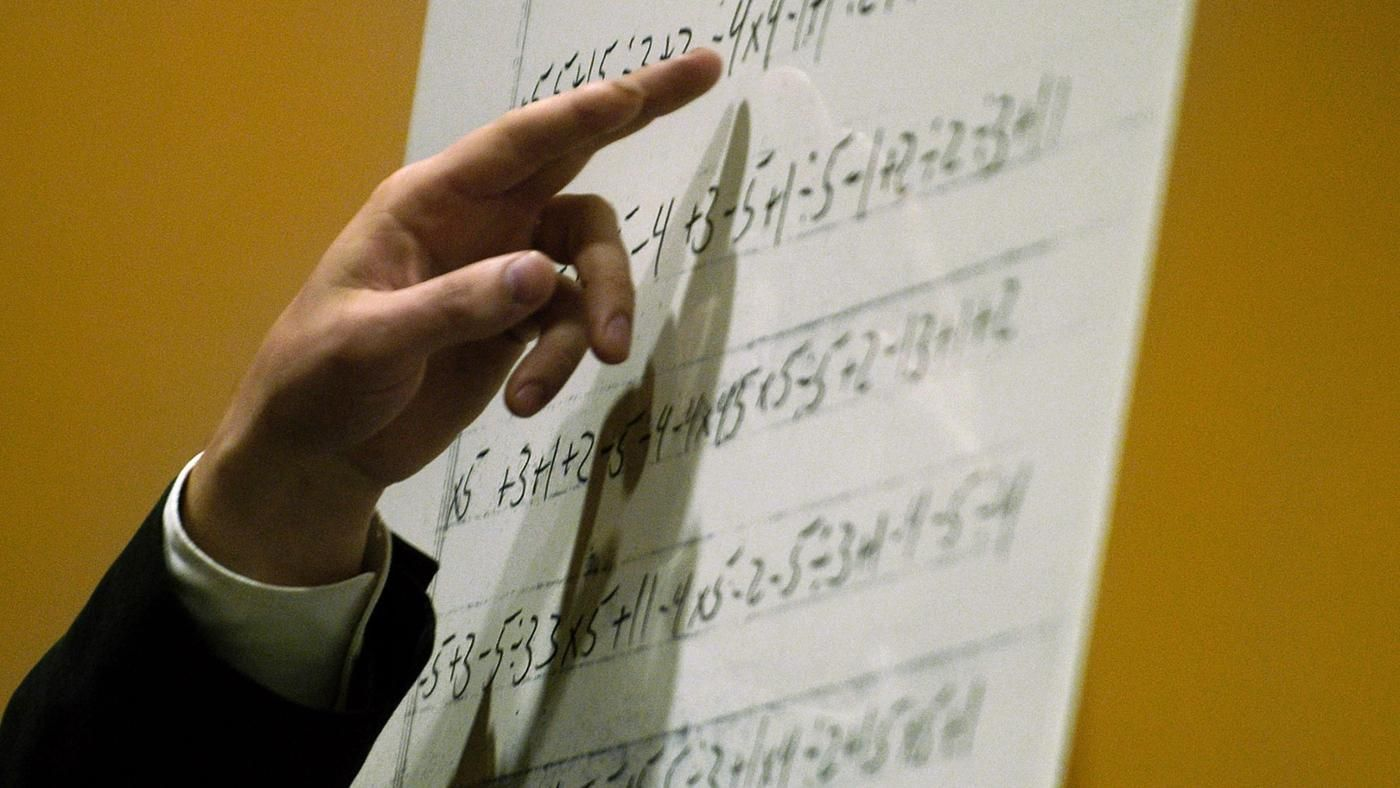Algebra

# Absolute Value: Level 4 Challenges

$\mathcal A=\displaystyle\sum_{i=1}^{2016}\left|x-a_i\right|$

Let $a_1,a_2,a_3,\ldots ,a_{2016}$ form an increasing arithmetic progression (AP) which consists of only positive terms. Let the minimum value of $\mathcal A$ be $2016^2$ for real $x$. Then find the sum of all possible values of the common difference of AP.

Notation: $| \cdot |$ denotes the absolute value function.

$\large x=|||\cdots |||2010-1|-2|-3|-\cdots -99|-100|$

What is the value of $x$?

For real $x$, find the minimum value of the expression below.

$|x-1| + |x-2| + \ldots + |x-100|$

Define a function $F$ as follows$F(w,x,y,z)=\dfrac { 1 }{ 8 } \left( S-T \right)$

where

$S=aw+bx+cy+dz-\left| w-x \right| -\left| aw+bx-cy-\left| w-x \right| \right|$
$T=\left| aw+bx+cy-dz-\left| w-x \right| -\left| aw+bx-cy-\left| w-x \right| \right| \right|$

For certain positive integers $a,b,c,d$ and for all $w,x,y,z$, this function $F\left(w,x,y,z\right)$ always returns the lowest of the values $w,x,y,z$. $\;\;$ For example

$F(4,-3,2,1)=-3$.

Let $a,b,c,d$ be integer digits of a $4$ digit integer $A = \overline{abcd}$. What is the value of $A$?

$\large |x - 1| + |2x-1| + |3x-1| + \cdots + |300x-1|$

If the minimum value of the expression above is $\dfrac AB$, and it occurs when $x = \dfrac CD$, where $(A, B)$ and $(C, D)$ are each coprime pairs of positive integers, find $A+B+C+D$.

×

Problem Loading...

Note Loading...

Set Loading...